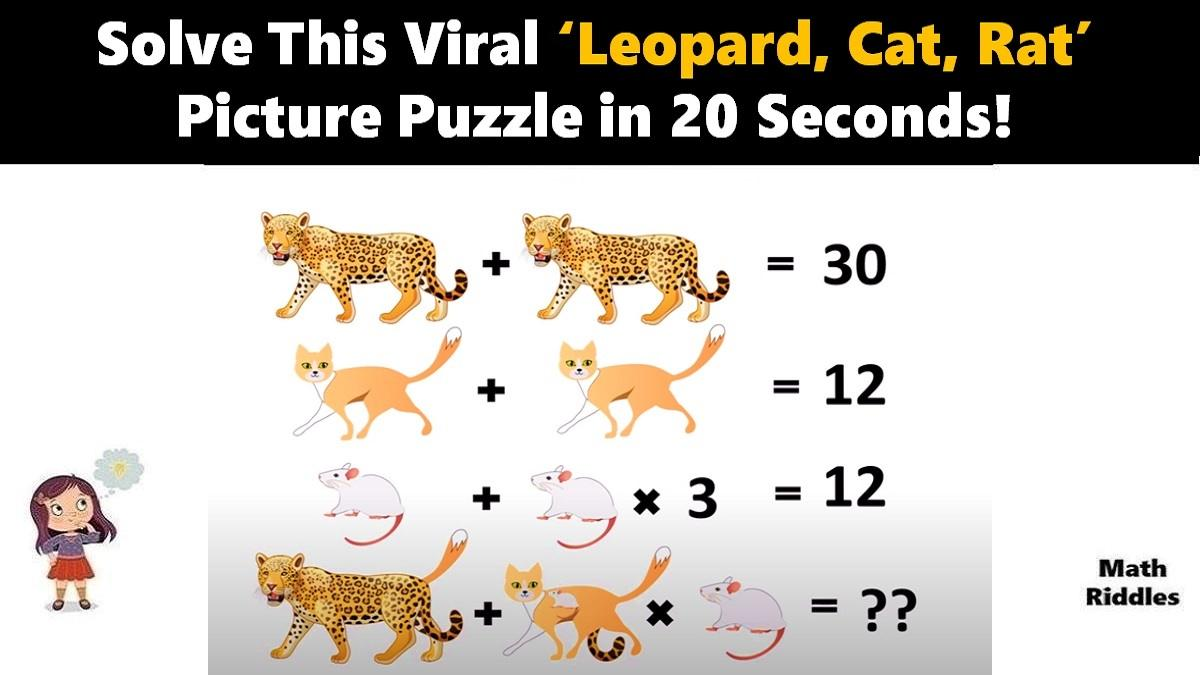# Math Riddles: Can You Solve This Viral ‘Leopard, Cat, Rat’ Picture Puzzle in 20 Seconds?

Math Picture Puzzles: Find out how brilliant you are. Only 1% of people with a high IQ can solve this tricky ‘Leopard, Cat, Rat’ math picture puzzle in 20 seconds.Math Riddles: Can You Solve This Viral ‘Leopard, Cat, Rat’ Picture Puzzle in 20 Seconds?

Math Riddles are so challenging, but that makes them worthwhile to solve. Math riddles are logical problems that require strong analytical abilities, high IQ, knowledge of math concepts, and good calculation skills. Math riddles have also been known to make Mathematics enjoyable for kids, students, and even adults to solve. Riddles, puzzles, and brain teasers can develop strategic thinking, logical reasoning, and problem-solving skills.

Today, we bring you another math picture puzzle that is all over social media. This only-for-genius ‘Leopard, Cat, Rat’ math picture puzzle has attracted more millions of answers but very few got it right.

Can you solve this ‘Leopard, Cat, Rat’ math picture puzzle? You have 20 seconds!

## Leopard, Cat, Rat Math Puzzle with Answers

Math Picture Puzzle #1

Row 1: There are two leopards that equals to 30.

1 Leopard + 1 Leopard = 30

=> 2 (Leopards) = 30

=> 1 Leopard = 30 / 2

=> 1 Leopard = 15

Now, we will use the value of 1 Leopard to solve the next rows of problems.

Row 2: Now, we have 2 Cats that equals to 12.

1 Cat + 1 Cat = 12

=> 2 (Cats) = 12

=> 1 Cat = 12 / 2

=> 1 Cat = 6

Now, we will use the value of 1 Cat to solve the next rows of problems.

Row 3: Let’s figure out the value of 1 Rat from this equation.

1 Rat + 1 Rat x 3 = 12

=> 1 Rat + 3 Rats = 12

=> 4 Rats = 12

=> 1 Rat = 12 / 4

=> 1 Rat = 3

Therefore, so far, we have solved that the value of 1 Leopard is 15, the value of 1 Cat is 6, and the value of 1 Rat is 3. Moving ahead, now we solve next row to figure out the final answer of this picture puzzle.

Row 4: Now, we reach the final question of the puzzle where we need to find out what the total of the final equation. Hence, we apply the values of each item that we have ascertained from the previous rows of this puzzle to find out the final sum.

Now, this is where 99% people fail to find the right answer. Notice, there is a tail of cat with the leopard and then there is a mouse as well as tail of leopard with the cat. We will break up the equation to get better understanding and application of values that we have ascertained in the previous equations.

(1 Leopard + 1 Cat Tail) + (1 Cat + 1 Rat + 1 Leopard Tail) x (1 Rat) = ?

=> (1 Leopard + 1 Cat) + (1 Cat + 1 Rat + 1 Leopard) x (1 Rat) = ?

=> (15 + 6) + (6 + 3 + 15) x (3) = ?

=> 21 + 24 x 3 = ?

=> Apply BODMAS

=> 21 + 72 = ?

=> 93

## Tell us in comments: Did you solve this math puzzle correctly in 20 seconds each?

Check out more amazing tricky math puzzles!

Also Read: Math Riddles: Can You Solve This Viral ‘Caterpillar, Clock, Flower’ Picture Puzzle in 20 Seconds?

Also Read: Math Riddles: Viral ‘Banana, Pineapple, Cherry, Orange’ Picture Puzzle, Only Genius Can Solve

Also Read: Math Riddles: Viral ‘Balloon’ Picture Puzzle, Only Genius Can Solve

Also Read: Math Riddles: Viral ‘Heart’ Picture Puzzle, Only Genius Can Solve